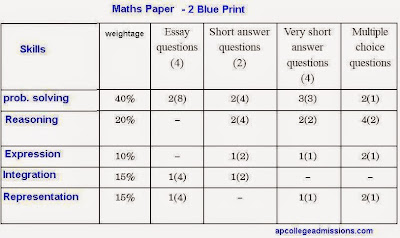## July 3, 2014

### 10th Class Maths New Model Papers for 2015 Exams in AP and Telangana

Here we are giving 10th Class / SSC / Tenth Class model question paper pattern and blue print of weightage for Maths subject. There are two papers for a total of 80 marks. The question papers are prepared by SCERT as per the new syllabus of Maths and new exam pattern for 2014-15 in AP and Telangana States.

SSC Maths Paper 1:

This paper consists of 4 marks, 2 marks and 1 mark questions.
1) 4 Marks Questions: 4 x 4 = 16 Marks
2) 2 Marks Questions: 2 x 6 = 12 Marks
3) 1 Mark Questions: 1 x 7 = 7 Marks
4) Objective type multiple choice questions: 10 x 1/2 = 5 Marks

SSC Maths Paper 2:

This paper also follows the same pattern as mentioned in Paper 1. There will be choice in four marks questions only for both the papers. Students will have to write answers for 4 questions out of 8 questions in four marks section.

Total time for the examination is Hrs 2 - 45 minutes. Out of these, 15 minutes will be given for the reading and understanding of the question paper and remaining time for answering the questions.